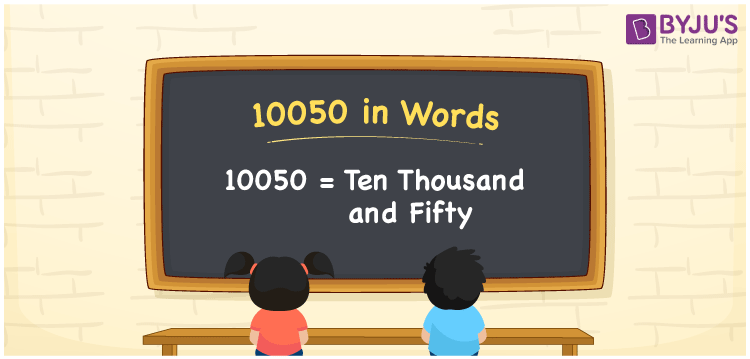# 10050 in Words

10050 in words can be written as Ten Thousand and Fifty. The fundamental concepts in Mathematics like counting or count can be learnt efficiently here. If you buy a bike for Rs. 10050, then you can say that “I bought a bike for Ten Thousand and Fifty Rupees”. To write numbers in words, the English alphabet is used. The numbers in words concept is explained here in a simple way to improve the conceptual knowledge of students. The 10050 can be read as “Ten Thousand and Fifty” in English.

 10050 in words Ten Thousand and Fifty Ten Thousand and Fifty in Numbers 10050

## 10050 in English Words## How to Write 10050 in Words?

Students can learn about the expanded form and the place value chart of 10050. Five digits are present in the number 10050. With the help of the place value chart given below, students will be able to understand the concepts with ease.

 Ten Thousands Thousands Hundreds Tens Ones 1 0 0 5 0

10050 can be written in expanded form as:

1 x Ten Thousand + 0 x Thousand + 0 × Hundred + 5 × Ten + 0 × One

= 1 x 10000 + 0 x 1000 + 0 × 100 + 5 × 10 + 0 × 1

= 10000 + 50

= 10050

= Ten Thousand and Fifty

Hence, 10050 in words is written as Ten Thousand and Fifty.

10050 is a natural number that precedes 10051 and succeeds 10049.

10050 in words – Ten Thousand and Fifty

Is 10050 an odd number? – No

Is 10050 an even number? – Yes

Is 10050 a perfect square number? – No

Is 10050 a perfect cube number? – No

Is 10050 a prime number? – No

Is 10050 a composite number? – Yes

## Frequently Asked Questions on 10050 in Words

### How to write 10050 in words?

10050 can be written in words as “Ten Thousand and Fifty”.

### How to write Ten Thousand and Fifty in numbers?

Ten Thousand and Fifty in numbers can be written as 10050.

### Is 10050 an odd or even number?

10050 is an even number as it is completely divisible by 2.